# Regression Analysis and Least Squares

March 29, 2018

In non-mathematical terminology, regression analysis involves fitting smooth curves to scattered data. Around 1800, determining the best method for regression analysis of the planets’ orbits was a major motivating factor for the development of the normal distribution , the central limit theorem , and the method of least squares . Regression analysis is relevant today for important data such as global surface temperature (Figure 3.17).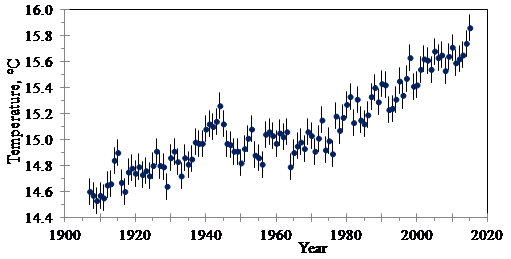Figure 3.17. Linear regression of global surface temperatures.

In its simplest form, linear regression fits a straight line to a series of data points (xnyn), n = 1, …, N, as Figure 3.17 illustrates. The equation for the line is y = ax + b, and we determine a and b. The error, or residual, is the difference between the line and data points at each xn: en = yn – axn – b.

### The Least Squares Method

The method of least squares determines the “best” values for a and b. This is achieved by summing the squares of the residuals, en, and finding the values of a and b that minimize the sum. Using calculus, the derivatives of the sum are set to zero with respect to a and b. Then, the two equations are solved for a and b. Equation 19 is the resulting formula:

(1)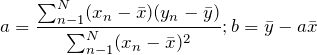(2)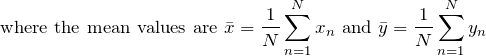Equation 19

To obtain the confidence interval for the curve fit, we can assume the errors are normally distributed. Equation 20 calculates the variance of the residuals. N – 2  is in the denominator because the two equations for a and b reduced the degrees of freedom by 2.

(3)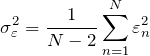Equation 20

Now, we must add the variance of the determination of the yn data, σyn2. It is often assumed that the error bound for measurement is equal to 2σy based on a 95% confidence level. The standard deviation for the line values, y, is:

(4)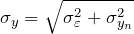Equation 21

The R-squared coefficient is a common measurement to determine the best fit for the regression analysis (Equation 22).

(5)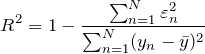Equation 22

The square root of R2 is often called the correlation coefficient,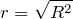. In the example of global surface temperatures (Figure 3.17), σe = 0.133º C. The error bound for each temperature measurement is ±0.1º C, so it is assumed that σyn= 0.050º C. Then, σy = 0.14º C, and the 95% confidence interval is ±0.28º C. The calculated value for R-squared is 0.83.

### Using Log Values for Linear Regression

In many cases, data on a linear scale does not fit a straight line. Instead of a non-linear curve, it is convenient to use logarithmic values for the linear regression. For example:

• log(y) vs. x
• y vs. log(x)
• log(y) vs. log(x)

#### Example

The damping in a structure causes the vibration to decay at the theoretical rate of A(t) = Ao e-2πf ζ t after impact. f is the frequency in Hz, t is the time in sec., and ζ  is the critical damping ratio.

To determine the damping value, the RMS acceleration level is measured versus time after impact (see Figure 3.18a for f = 200Hz.)

The theoretical decay will be a straight line when plotted as log(A) = log(Ao) – [2π  f ζ  log(e)] t (Figure 3.18b). In this example, the linear regression equates the slope at -8.28, so ζ = 8.28 / [2π f  log(e)] = 0.015.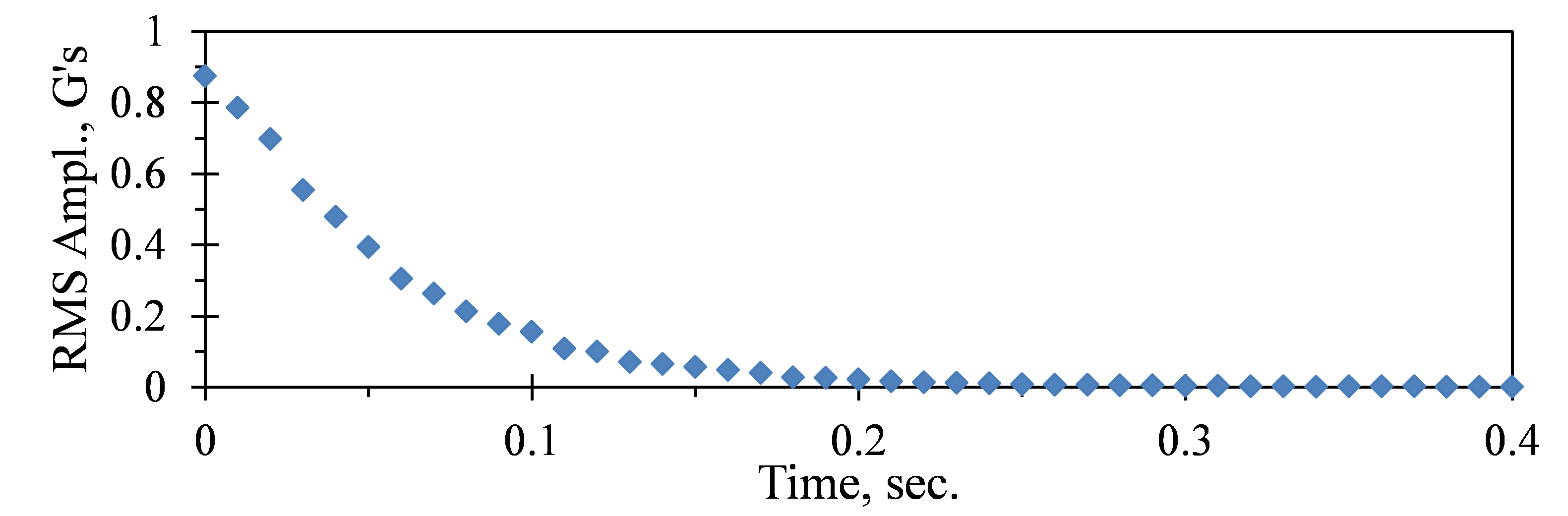Figure 3.18a. RMS acceleration level measured vs. time after the structure is impacted for f = 200Hz.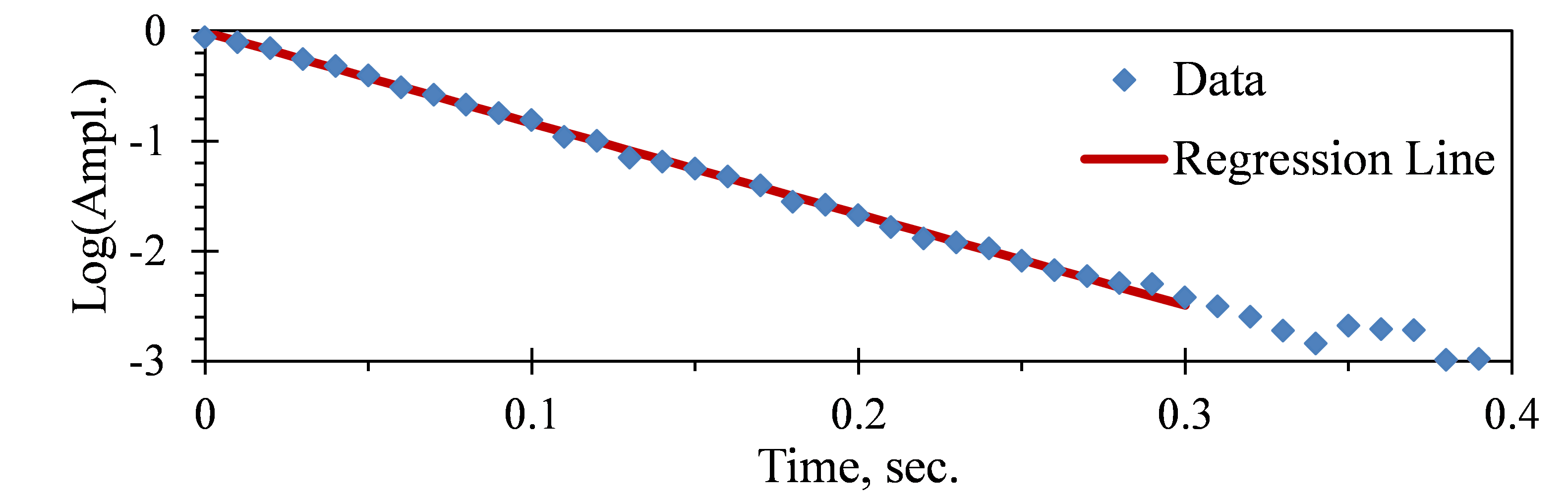Figure 3.18b. Regression analysis of transient vibration decay.